Saturday, September 25, 2021
HomeOscillatorsOscillators - Classification, How it Works, Barkhausen Criterian, Advantage

# Oscillators – Classification, How it Works, Barkhausen Criterian, Advantage

Oscillator is an electronic circuit which helps in converting DC to AC. This post will discuss about what is Oscillator, its various types, how it works, Barkhausen Criterian, its applications, advantages and disadvantages.

## What is an Oscillator

Oscillators are electronic circuits that generate output signal either in the form of sinusoidal, non-sinusoidal depending on the type of Oscillators used.Fig. 1 – Introduction to Oscillator

It converts DC to AC or periodic waveform. It’s circuits are basically used in watches, computer clocks, radio receivers etc. This Circuits work on the principle of positive feedback and regenerative feedback. The operating frequency ranges from less than 1 cycle/ sec to billions of cycles/ sec.

Fig. 2 shows the basic block diagram of Oscillator which consists of oscillatory circuit, amplifier and a feedback network. Oscillatory circuit can be RC Network, LC Network or quartz. Amplifier converts DC to AC. The output of the amplifier is provided back to the Oscillatory circuit through Feedback Network. The oscillations generated by Oscillatory circuit acts as input to the amplifier.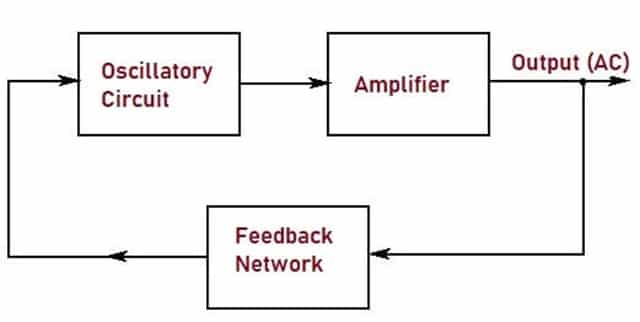Fig. 2 – Block Diagram of Oscillatory Circuit

## Barkhausen Criterion

It is defined as the necessary criterion for any Electronic Circuit to behave as an Oscillator. Barkhausen Criterion states that:

• Magnitude of overall gain around the loop is unity
• Overall phase shift around the loop is either zero or multiple of 360 degree.

## Types of Oscillator

• Feedback (Harmonic) Oscillators
• Relaxation Oscillators

### Feedback (Harmonic) Oscillators

Feedback Oscillators are also called Harmonic Oscillators. In this type of Oscillatory circuit, fraction of the output is returned to the input without phase shift. Feedback Oscillatory circuit consists of an Amplifier and positive feedback circuit which provides gain and produces phase shift resulting in attenuation. These Oscillatory circuits are used to generate sinusoidal waveforms. They are of two types. They are:

• RC Oscillator
• LC Oscillator

#### RC Oscillator

This circuit involves Resistors and Capacitors and types of RC Oscillators are:

• Wien Bridge Oscillator
• Phase Shift Oscillator
##### Wien Bridge Oscillator

The output of the amplifier is applied between the terminals a and c as shown in the Fig.3, which acts as the input to the feedback network. While the amplifier input is supplied from the diagonal terminals b and d, which is the output from the feedback network. Thus, amplifier supplies its own input through the Wien Bridge feedback network.

Resistors, Capacitors which are connected in series and parallel decide the frequency of the Oscillator and is called as lead-lag network. At lower frequencies, the network leads and at higher frequencies, the network lags.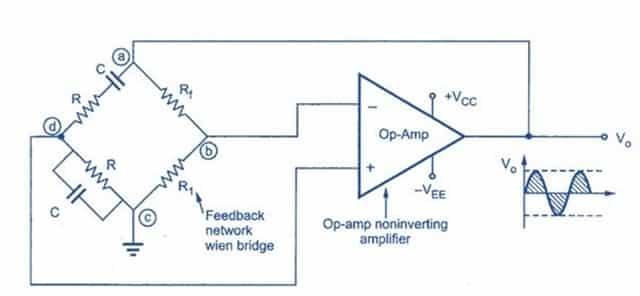Fig. 3 – Wien Bridge Oscillator

##### Phase Shift Oscillator

This circuit uses inverting amplifier and three high-pass filter circuits. It produces positive feedback and required phase shift. The output of inverting amplifier acts as input to the feedback network. The output signal from the feedback Network is input to the amplifier and thus loop is established with 3600phase shift generating Oscillations.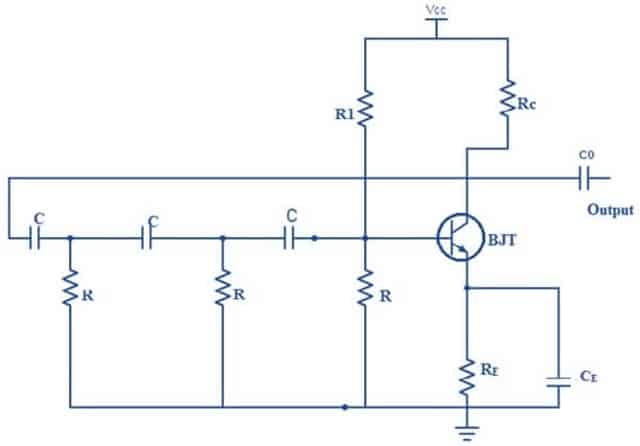Fig. 4 – Phase Shift Oscillator

#### LC Oscillator

This circuit involves Inductors and Capacitors. The types of LC Oscillators are:

• Hartley Oscillator
• Colpitts Oscillator
##### Hartley Oscillator

The frequency of oscillations in this type of Oscillator is determined by the tuned circuit, consisting of inductors and capacitors. Fig. 5 shows the circuit diagram of Hartley Oscillatory circuit in which Rc is collector resistor and Re represents Emitter Resistance. R1 and R2 resistors form voltage divider network. This circuit is similar to common-emitter amplifier configuration.

When the power is switched on, the transistor starts to conduct which increases collector current. This current helps in charging the Capacitors. Once they are charged to maximum, the capacitors starts to discharge through inductors L1 and L2. The charging and discharging cycles produce oscillations in the Tank circuit. Oscillation current produces AC voltage which is 180 degree out of phase.

Output of the amplifier acts as input across inductor L1 and feedback voltage from L2 is applied to the base of transistor. The output produced is in-phase with the voltage of Tank circuit. This signal will be amplified which has a net phase-shift of 360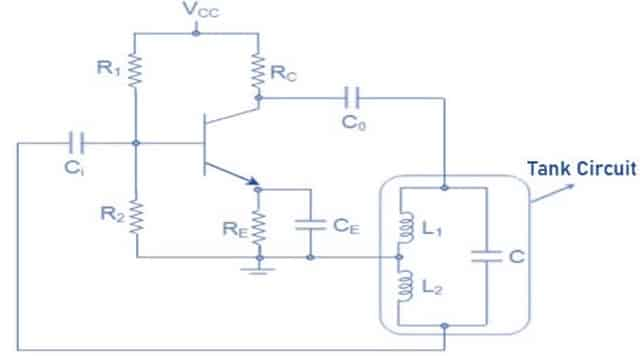Fig. 5 – Hartley Oscillator

##### Colpitts Oscillator

This circuit consists of single stage inverting amplifier and an L-C phase shift network. It is similar to common collector configuration. Capacitors C1 and C2 which are connected in series form the potential divider network which helps in providing feedback voltage. Collector supply voltage Vcc is applied to the collector of transistor through a resistance RC which allows maximum flow of current through the transistor. Coupling capacitor Cc in the output circuit prevents dc current flow to the tank circuit.

The output of the phase-shift L-C network is coupled from the junction of L and C2 to the amplifier input at base through coupling capacitor CC, which prevents dc but provides path to ac. Transistor itself produces a phase shift of 180° and capacitive feedback provides another phase shift of 180°. Thus, a net phase shift of 360° is obtained which is necessary for generating oscillations.

The oscillations are applied to Base-Emitter junction of the transistor and is amplified. The amplified output is fed to the tank circuit which ensures undamped oscillations.Fig. 6 – Colpitts Oscillator

### Relaxation Oscillators

This circuit uses a RC timing circuit driven by Schmitt trigger to generate a waveform. The resulting signal is either a square wave or non-sinusoidal waveform.

## How does Oscillator Work

To understand the working principle of Oscillator, let us consider Feedback Oscillatory Circuit as shown in the fig. As we know that in this circuit, part of the output voltage is returned to the input which is in-phase. This in-phase Feedback voltage is amplified to generate the output voltage and a part of this voltage acts as input to the feedback circuit. This circuit generates feedback voltage which is fed back to input. This creates a loop and sinusoidal output waveform is obtained. This is called as Oscillation.

In the below circuit, S is switch and A is amplifier. No Oscillation occurs when switch is open. When the circuit is closed, if Vi = input voltage, then output voltage V0 = AvVi and βV0= Vf be the voltage that is fed back to the circuit. If Vf = Vi, then the output voltage continues to exist even in the absence of input voltage.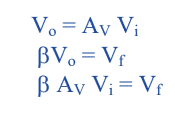βAv is called as Loop Gain and is equal to unity. Hence, we can conclude that when input voltage is removed from a closed circuit, Oscillations can be generated at the output if Loop Gain is equal to 1.

##Fig. 7 – Representation of Working Principle

## Applications of Oscillator

The applications include:

• They are used as sweep circuits.
• They are used to generate clocks in digital systems.
• They are extensively used in computers, Counters, Timers, oscilloscopes.

• Wien Bridge Oscillator produces highly stable and low distorted output.
• Modification of Frequency of Oscillation is possible.
• Less noise is produced.
• They can be designed at low cost.

• Colpitts Oscillator is hard to design.
• Frequency stability of phase shift Oscillator is poor.
• The amplitude of oscillations of LC circuit is difficult to control.
• Hartley Oscillator cannot be used as low frequency oscillator as the value and size of inductors become large.

Recommended Watch:

```Also Read:
Slew Rate for OP-AMP, Square and Sinusoidal Wave - How to Calculate It
Power Factor - Power Triangle, Types, PFC, Applications, Advantages
Voltmeter - Working Principle, Voltage Sensitivity, Types and Applications
Transistor - Classification, Configuration, Applications, Advantages```Laxmi Ashrit
Laxmi is a B.E (Electronics & Communication) and has work experience in RelQ Software as Test Engineer and HP as Technical support executive. She is an author, editor and partner at Electricalfundablog.
RELATED ARTICLES